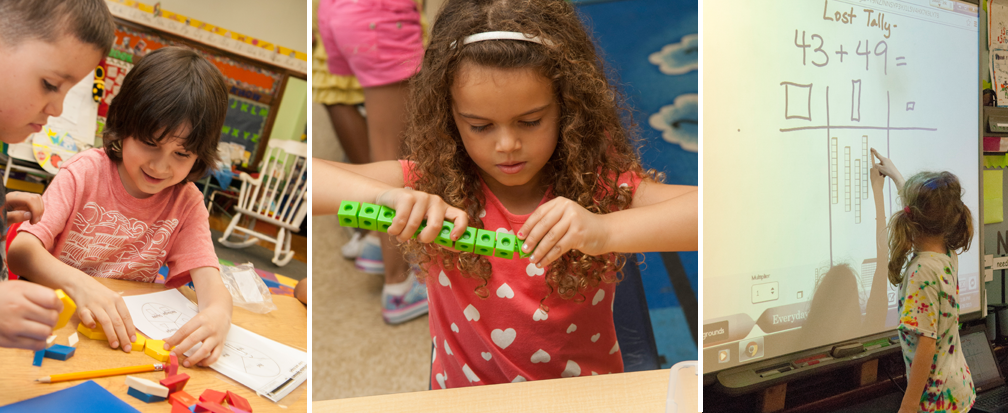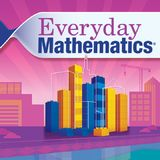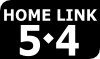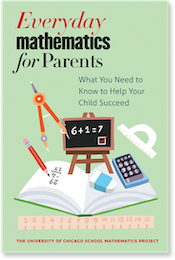### Select a Unit

• Unit 1 Place Value; Multidigit Addition and Subtraction
• Unit 2 Multiplication and Geometry
• Unit 3 Fractions and Decimals
• Unit 4 Multidigit Multiplication
• Unit 5 Fraction and Mixed-Number Computation; Measurement
• Unit 6 Division; Angles
• Unit 7 Multiplication of a Fraction by a Whole Number; Measurement
• Unit 8 Fraction Operations; Applications

### Finding the Unit and Lesson Numbers

Everyday Mathematics is divided into Units, which are divided into Lessons. In the upper-left corner of the Home Link, you should see an icon like this:The Unit number is the first number you see in the icon, and the Lesson number is the second number. In this case, the student is working in Unit 5, Lesson 4. To access the help resources, you would select "Unit 5" from the list above, and then look for the row in the table labeled "Lesson 5-4."The University of Chicago School Mathematics Project

University of Chicago Press